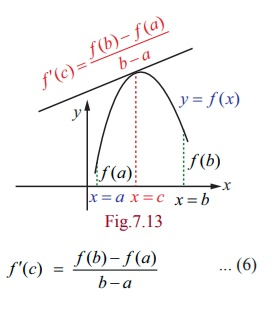Home | | Maths 12th Std | Mean Value Theorem

# Mean Value Theorem

Mean value theorem establishes the existence of a point, in between two points, at which the tangent to the curve is parallel to the secant joining those two points of the curve.

Mean Value Theorem

Mean value theorem establishes the existence of a point, in between two points, at which the tangent to the curve is parallel to the secant joining those two points of the curve. We start this section with the statement of the intermediate value theorem as follows :

Theorem 7.1 (Intermediate value theorem)

If f is continuous on a closed interval [a , b] , and c is any number between f (a) and f (b) inclusive, then there is at least one number x in the closed interval [a , b] , such that f ( x) = c .

## RolleŌĆÖs Theorem

Theorem 7.2 (RolleŌĆÖs Theorem)

Let f ( x) be continuous on a closed interval [a , b] and differentiable on the open interval (a , b)

If f (a) = f (b) , then there is at least one point c Ōłł (a, b) where fŌĆÖ(c) =0.Geometrically this means that if the tangent is moving along the curve starting at x = a towards as in FigŌĆē7.2 x = b then there exists a c Ōłł ( a, b) at which the tangent is parallel to the x -axis.

## LagrangeŌĆÖs Mean Value Theorem

Theorem 7.3

Let f (x) be continuous in a closed interval [a, b] and  differentiable in the open interval (a , b) (where f (a), f (b) are not necessarily equal). Then there exist at least one point c Ōłł( a , b) such that,

f ŌĆ▓(c) = f (b) ŌłÆ f (a) / b ŌłÆ a ... (6)Remark

If f (a) = f (b) then LagrangeŌĆÖs Mean Value Theorem gives the RolleŌĆÖs theorem. It is also known as rotated RolleŌĆÖs Theorem.

Remark

A physical meaning of the above theorem is the number f (b) ŌłÆ f (a) / b ŌłÆ a =can be  thought of as the average rate of change in f ( x) over (a, b) and f (c) as an instantaneous change.

A geometrical meaning of the LagrangeŌĆÖs mean value theorem is that the instantaneous rate of change at some interior point is equal to the average rate of change over the entire interval. This is illustrated as follows :

If a car accelerating from zero takes just 8 seconds to travel 200 m, its average velocity for the 8 second interval is 200/8 = 25 m/s. The Mean Value Theorem says that at some point during the travel the speedometer must read exactly 90 km/h which is equal to 25 m/s.

Theorem 7.4

If f ( x) is continuous in closed interval [a , b] and differentiable in open interval (a , b) and if f ŌĆ▓( x) > 0, ŌłĆx Ōłł (a, b) , then for, x1 , x2 Ōłł[a , b] , such that x1 < x2 we have, f (x1 ) < f (x2 ) .

Proof

By the mean value theorem, there exists a c Ōłł ( x1 , x2 ) ŌŖé (a, b) such that,

f (x2 ) ŌłÆ f (x1)  /  x2 ŌłÆ x1 = f ŌĆ▓(c)

Since f ŌĆ▓(c) > 0 , and x2 ŌłÆ x1 > 0 we have fŌĆē(x2) ŌĆō fŌĆē(x1) > 0.

We conclude that, whenever x1 < x2 , we have f (x1 ) < f (x2 ) .

Remark

If f ŌĆ▓( x) < 0, ŌłĆx Ōłł (a, b) , then for, x1 , x2 Ōłł [a, b] , such that x1 < x2 we have, f (x1 ) > f (x2 ) .

The proof is similar.

Tags : Differential Calculus | Mathematics , 12th Maths : UNIT 7 : Applications of Differential Calculus
Study Material, Lecturing Notes, Assignment, Reference, Wiki description explanation, brief detail
12th Maths : UNIT 7 : Applications of Differential Calculus : Mean Value Theorem | Differential Calculus | Mathematics